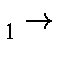### Franck-Condon considerations

The Frank-Condon principle states that electronic transitions take place in times that are very short compared to the time required for the nuclei to move significantly. Because of this, care must be taken to ensure that the calculations actually do reflect what is wanted.

Examples of various phenomena which can be studied are:

Photoexcitation
If the purpose of a calculation is to predict the energy of photoexcitation, then the ground-state should first be optimized. Once this is done, then a C.I. calculation can be carried out using 1SCF. With the appropriate keywords (MECI C.I.=n etc.), the energy of photoexcitation to the various states can be predicted.

A more expensive, but more rigorous, calculation would be to optimize the geometry using all the C.I. keywords. This is unlikely to change the results significantly, however.

Fluorescence
If the excited state has a sufficiently long lifetime, so that the geometry can relax, then if the system returns to the ground state by emission of a photon, the energy of the emitted photon will be less (it will be red-shifted) than that of the exciting photon. To do such a calculation, proceed as follows:
• Optimize the ground-state geometry using all the keywords for the later steps, but specify the ground state, e.g. MECI C.I.=3 GNORM=0.01 .
• Optimize the excited state, e.g. C.I.=3 ROOT=2 GNORM=0.01 MECI.
• Calculate the Franck-Condon excitation energy, using the results of the ground-state calculation only.
• Calculate the Franck-Condon emission energy, using the results of the excited state calculation only.
• If indirect emission energies are wanted, these can be obtained from the ΔHf of the optimized excited and optimized ground-state calculations.
In order for fluorescence to occur, the photoemission probability must be quite large, so only transitions of the same spin are allowed. For example, if the ground state is S0, then the fluorescing state would be S1.
Phosphorescence
If the photoemission probability is very low, then the lifetime of the excited state can be very long (sometimes minutes). Such states can become populated by ST1 intersystem crossing. Of course, the geometry of the system will relax before the photoemission occurs.
Indirect emission
If the system relaxes from the excited electronic, ground vibrational state to the ground electronic, ground vibrational state, then a more complicated calculation is called for. The steps of such a calculation are:
• Optimize the geometry of the excited state.
• Using the same keywords, except that the ground state is specified, optimize the geometry of the ground state.
• Take the difference in ΔHf of the optimized excited and optimized ground-state calculations.
• Convert this difference into the appropriate units.
Excimers
An excimer is a pair of molecules, one of which is in an electronic excited state. Such systems are usually stabilized relative to the isolated systems. Optimization of the geometries of such systems is difficult. Suggestions on how to improve this type of calculation would be appreciated.